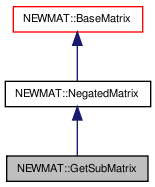# NEWMAT::GetSubMatrix Class Reference

`#include <newmat.h>`

Inheritance diagram for NEWMAT::GetSubMatrix:[legend]

## Detailed Description

Definition at line 1558 of file newmat.h.

List of all members.

## Public Member Functions

GetSubMatrix (const GetSubMatrix &g)
~GetSubMatrix ()
GeneralMatrixEvaluate (MatrixType mt=MatrixTypeUnSp)
void operator= (const BaseMatrix &)
void operator+= (const BaseMatrix &)
void operator-= (const BaseMatrix &)
GetSubMatrixoperator= (const GetSubMatrix &m)
void operator<< (const BaseMatrix &)
void operator<< (const Real *)
void operator<< (const int *)
MatrixInput operator<< (Real)
MatrixInput operator<< (int f)
void operator= (Real)
void operator+= (Real)
void operator-= (Real r)
void operator*= (Real)
void operator/= (Real r)
void Inject (const GeneralMatrix &)
MatrixBandWidth BandWidth () const

## Private Member Functions

GetSubMatrix (const BaseMatrix *bmx, int rs, int rn, int cs, int cn, bool is)
void SetUpLHS ()

int row_skip
int row_number
int col_skip
int col_number
bool IsSym

class BaseMatrix

## Constructor & Destructor Documentation

 NEWMAT::GetSubMatrix::GetSubMatrix ( const BaseMatrix * bmx, int rs, int rn, int cs, int cn, bool is ) ` [private]`

Definition at line 1567 of file newmat.h.

 NEWMAT::GetSubMatrix::GetSubMatrix ( const GetSubMatrix & g )

Definition at line 1573 of file newmat.h.

 NEWMAT::GetSubMatrix::~GetSubMatrix ( )

Definition at line 1576 of file newmat.h.

## Member Function Documentation

 MatrixBandWidth NEWMAT::GetSubMatrix::BandWidth ( ) const` [virtual]`

Reimplemented from NEWMAT::NegatedMatrix.

Definition at line 530 of file newmat4.cpp.

 GeneralMatrix * NEWMAT::GetSubMatrix::Evaluate ( MatrixType mt = `MatrixTypeUnSp` ) ` [virtual]`

Reimplemented from NEWMAT::NegatedMatrix.

Definition at line 276 of file newmat5.cpp.

 void NEWMAT::GetSubMatrix::Inject ( const GeneralMatrix & gmx )

Definition at line 210 of file submat.cpp.

 void NEWMAT::GetSubMatrix::operator*= ( Real r )

Definition at line 313 of file submat.cpp.

Referenced by operator/=().

 void NEWMAT::GetSubMatrix::operator+= ( Real r )

Definition at line 288 of file submat.cpp.

 void NEWMAT::GetSubMatrix::operator+= ( const BaseMatrix & bmx )

Definition at line 228 of file submat.cpp.

Referenced by operator-=().

 void NEWMAT::GetSubMatrix::operator-= ( Real r )

Definition at line 1589 of file newmat.h.

 void NEWMAT::GetSubMatrix::operator-= ( const BaseMatrix & bmx )

Definition at line 258 of file submat.cpp.

 void NEWMAT::GetSubMatrix::operator/= ( Real r )

Definition at line 1591 of file newmat.h.

 MatrixInput NEWMAT::GetSubMatrix::operator<< ( int f )

Definition at line 1880 of file newmat.h.

 MatrixInput NEWMAT::GetSubMatrix::operator<< ( Real f )

Definition at line 411 of file newmat5.cpp.

 void NEWMAT::GetSubMatrix::operator<< ( const int * r )

Definition at line 178 of file submat.cpp.

 void NEWMAT::GetSubMatrix::operator<< ( const Real * r )

Definition at line 161 of file submat.cpp.

 void NEWMAT::GetSubMatrix::operator<< ( const BaseMatrix & bmx )

Definition at line 98 of file submat.cpp.

 void NEWMAT::GetSubMatrix::operator= ( Real r )

Definition at line 195 of file submat.cpp.

 GetSubMatrix& NEWMAT::GetSubMatrix::operator= ( const GetSubMatrix & m )

Definition at line 1581 of file newmat.h.

Referenced by operator=().

 void NEWMAT::GetSubMatrix::operator= ( const BaseMatrix & bmx )

Definition at line 126 of file submat.cpp.

 void NEWMAT::GetSubMatrix::SetUpLHS ( ) ` [private]`

Definition at line 83 of file submat.cpp.

Referenced by Inject(), operator*=(), operator+=(), operator-=(), operator<<(), and operator=().

## Friends And Related Function Documentation

 friend class BaseMatrix` [friend]`

Reimplemented from NEWMAT::NegatedMatrix.

Definition at line 1571 of file newmat.h.

## Member Data Documentation

 int NEWMAT::GetSubMatrix::col_number` [private]`
 int NEWMAT::GetSubMatrix::col_skip` [private]`
 bool NEWMAT::GetSubMatrix::IsSym` [private]`

Definition at line 1564 of file newmat.h.

Referenced by Evaluate().

 int NEWMAT::GetSubMatrix::row_number` [private]`
 int NEWMAT::GetSubMatrix::row_skip` [private]`

The documentation for this class was generated from the following files:

 newmat11b Generated Mon May 9 04:54:19 2016 by Doxygen 1.6.3